# 【带着canvas去流浪（4）】绘制散点图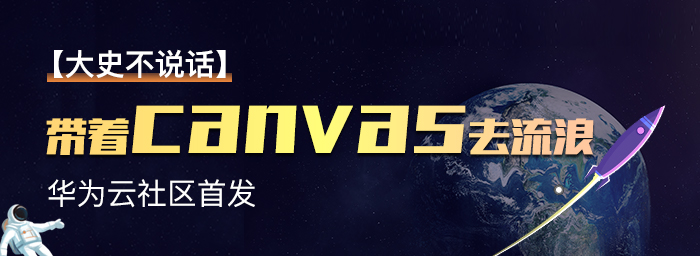## 一. 任务说明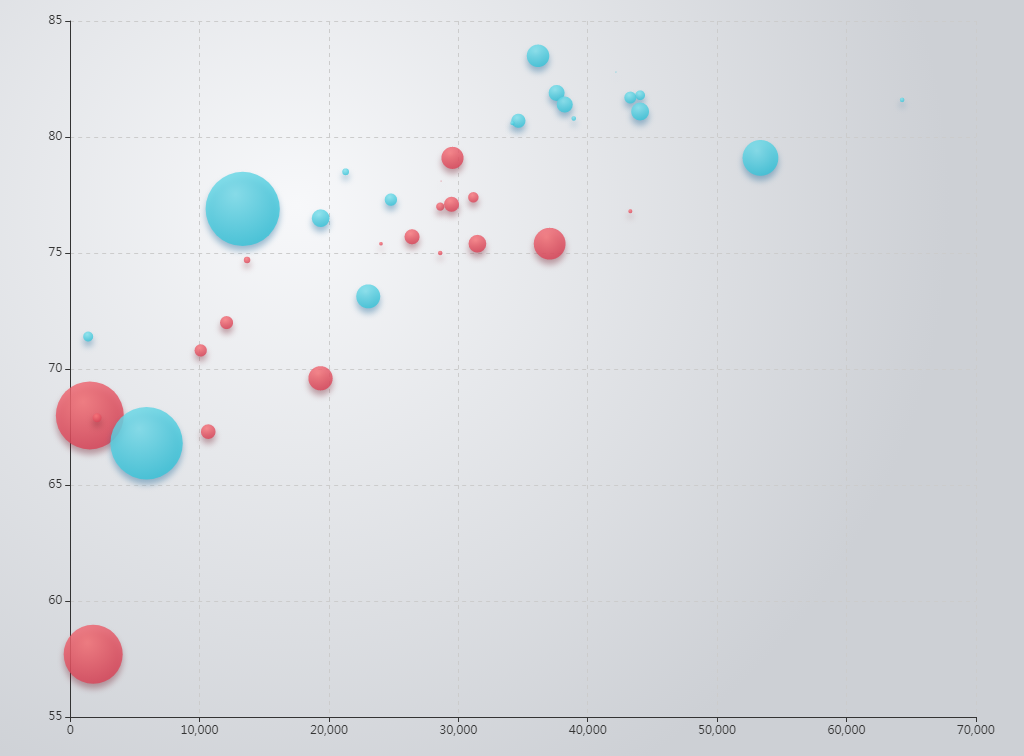## 二. 重点提示

//求散点半径时所使用的公式
//1.直接数值
r = value * 5 / 100000000;
//2.求对数
r = Math.log(value);
//3.求指数
r = Math.pow(value,0.4) / 100;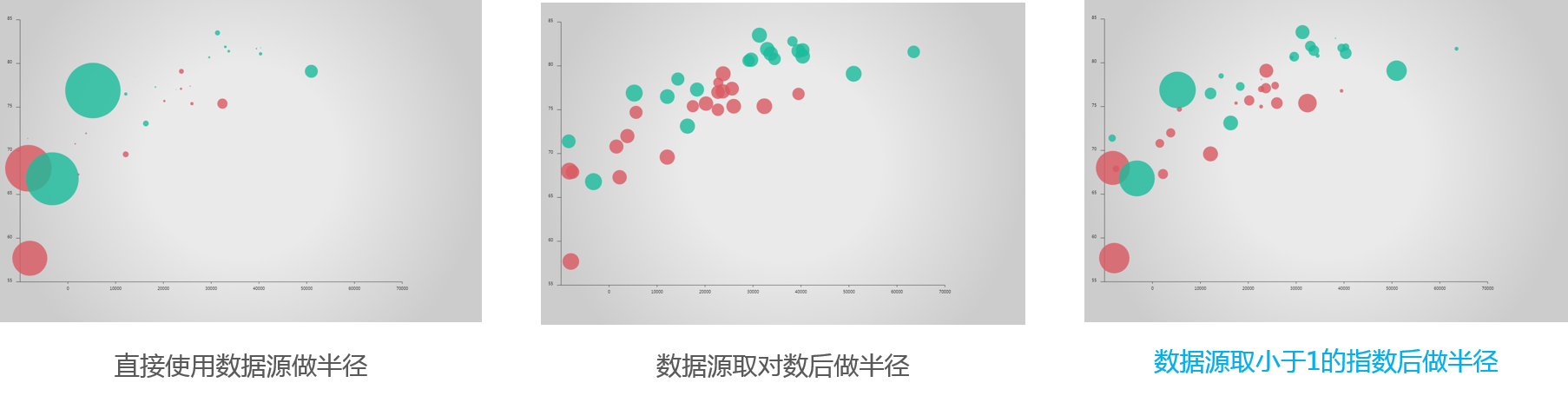坐标映射的实现思路其实并不算复杂，它的概念可以参考算法的时间复杂度来进行理解，挑选一个增长更快的映射函数来区分相近的点，或者挑选一个增长更慢的映射函数来减小大跨度数据之间的差异，在数据可视化中是非常实用的技巧。本文示例中的效果是笔者自己手动调的，如果要实现根据数据集自动挑选适当的映射函数，还需要设计一些计算方法，感兴趣的读者可以自行研究。

## 三. 示例代码

/*数据点来自于百度Echarts官方示例库，每个数值分别表示[横坐标，纵坐标，数值，国家，年份]
*[28604,77,17096869,'Australia',1990]
*/

/**
* 绘制数据
*/
function drawData(options) {
let data = options.data;//获取数据集
let xLength = (options.chartZone - options.chartZone);
let yLength = (options.chartZone - options.chartZone);
let gap = xLength / options.xAxisLabel.length;

//遍历两个年份
for(let i = 0; i < data.length ;i++){
let x,y,r,c;
context.fillStyle = options.colorPool[i];//从颜色池中选取颜色
context.globalAlpha = 0.8;//为避免点覆盖，采取半透明绘制
//遍历各个数据点
for(let j = 0; j < data[i].length ; j++){
//计算坐标
x = options.chartZone + xLength * data[i][j] / 70000;
y = options.chartZone - yLength * (data[i][j] - 55) / (85 - 55);

//直接数值
r = data[i][j] * 5 / 100000000;
//求对数
r = Math.log(data[i][j]);
//开根号
r = Math.pow(data[i][j],0.4) / 100;
//绘制散点
context.beginPath();
context.arc(x, y , r , 0 , 2*Math.PI,false);
context.fill();
context.closePath();
}
}
}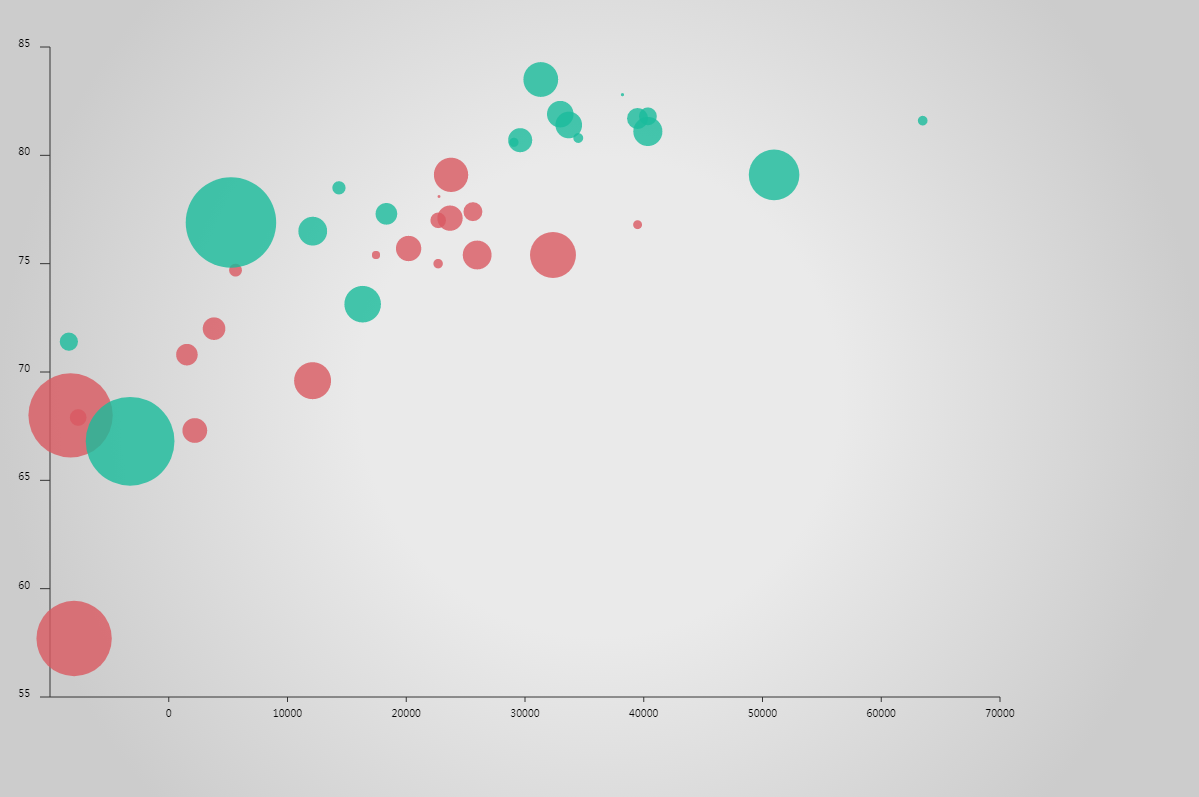## 四.散点hover交互效果的实现

### 4.1 基本算法

1. canvas元素上监听鼠标移动事件，将鼠标坐标转换为canvas坐标系的坐标值。
2. 遍历数据点查看是否存在当前鼠标点距离某个数据中心点的距离小于其散点的绘制半径，如果有则认为鼠标在该点之上。
3. 利用之前缓存的该点绘图数据，调整绘图样式，增大数据点的绘图半径覆盖式绘图即可。
4. 当鼠标距离任何数据点的距离都大于该点的绘图半径，或鼠标从一个hover数据点移动到另一个hover点时，均需要调用一次resetHover( )方法清除之前的hover状态。
5. 为了恢复hover前的状态，可以使用【离屏canvas技术】缓存首次绘图后的结果，然后使用drawImage( )方法将对应区域恢复到hover前的状态。

### 4.2 参考代码

hover效果的关键代码如下，完整示例代码请在demo中获取，或访问【我的github仓库】

/*简单hover效果*/
canvas.onmousemove = function (event) {
//转换鼠标坐标为相对canvas
let pos = {
x: event.clientX - rect.left,
y: event.clientY - rect.top
}
//获取当前hover点坐标
let hoverPoint = checkHover(options, pos);
/**
* 如果当前有聚焦点
*/
if (hoverPoint) {
//如果当前点和上一次记录的hover点是不同的点，则先调一次reset方法，然后把hover点更改为当前的点
let samePoint = options.hoverData === hoverPoint ? true : false;
if (!samePoint) {
resetHover();
options.hoverData = hoverPoint;
}
//绘制当前点的hover状态
paintHover();
} else{
//第一次尝试手动恢复
// resetHover();
//使用离屏canvas恢复
resetHoverWithOffScreen();
}
}

/*检测是否hover在散点之上*/
function checkHover(options,pos) {
let data = options.paintingData;
let found = false;
for(let i = 0; i < data.length; i++){
found = false;
for(let j = 0; j < data[i].length; j++){
if (Math.sqrt(Math.pow(pos.x - data[i][j].x , 2) + Math.pow(pos.y - data[i][j].y , 2)) < data[i][j].r) {
found = data[i][j];
break;
}
}
if (found) break;
}
return found;
}

/*绘制hover状态*/
function paintHover() {
let {x,y,r,c} = options.hoverData;
let step = 0.5;
context.globalAlpha = 1;
context.fillStyle = c;
//逐帧增加hover点的绘图半径，重新绘制hover状态的散点
for(let i = 0 ; i < 30; i++){
context.beginPath();
context.arc(x,y,r + i * step, 0 , 2*Math.PI,false);
context.fill();
context.closePath();
}
}

/*首次尝试的取消高亮状态的函数*/
function resetHover() {
if (!options.hoverData) return;
let {x,y,r,c} = options.hoverData;
let step = 0.5;
context.globalAlpha = 1;
for(let i = 29; i>0; i--){
context.save();
//绘制外圆范围
context.beginPath();
context.arc(x,y,r + 30 * step, 0 , 2*Math.PI,false);
context.closePath();
//设置剪裁区域
context.clip();
//用全局背景色绘制剪裁区背景
context.globalAlpha = 1;
context.fillStyle = options.globalGradient;
context.fill();
//绘制内圆
context.beginPath();
context.arc(x,y,r + i * step, 0 , 2*Math.PI,false);
context.closePath();
context.fillStyle = c;
context.globalAlpha = 0.8;
//填充内圆
context.fill();
context.restore();
}
options.hoverData = null;
console.log('清除hover效果');
}

//利用离屏canvas恢复hover前的状态
function  resetHoverWithOffScreen() {
if (!options.hoverData) return;
let {x,y,r,c} = options.hoverData;
let step = 0.5;
context.globalAlpha = 1;
for(let i = 29; i>0; i--){
context.save();
//将hover状态下数据点圆所在的正方形范围恢复为hover前的状态
context.drawImage(canvas2, x - r - 30 * step, y - r - 30 * step , 2 * (r + 30 * step),2*(r + 30 * step),x - r - 30 * step, y - r - 30 * step , 2*(r + 30 * step),2*(r + 30 * step));
//绘制内圆
context.beginPath();
context.arc(x,y,r + i * step, 0 , 2*Math.PI,false);
context.closePath();
context.fillStyle = c;
context.globalAlpha = 0.8;
//填充内圆
context.fill();
context.restore();
}
options.hoverData = null;
console.log('清除hover效果');
}


### 4.3 Demo中的小问题

1. 为了简化代码，demo中的一些绘图数据并没有参数化，而是采取直接写死的形式放在代码里，尤其是逐帧绘图的代码，一般开发中此处都会配合动画来进行实现。

2. 为了重置某个数据点的hover状态，笔者最初的实现思路是在每一帧中，使用context.clip( )方法裁切出绘图区域，先用全局背景绘制出背景图，缩小数据点半径，然后再绘制数据点，直到半径缩小至hover前的值。但在实现后发现这种方式存在一个问题，那就是数据点之间出现重叠时，如果只是简单地背景重绘，就会将部分重叠区域清除掉，造成其他数据点无法复原，如下图所示：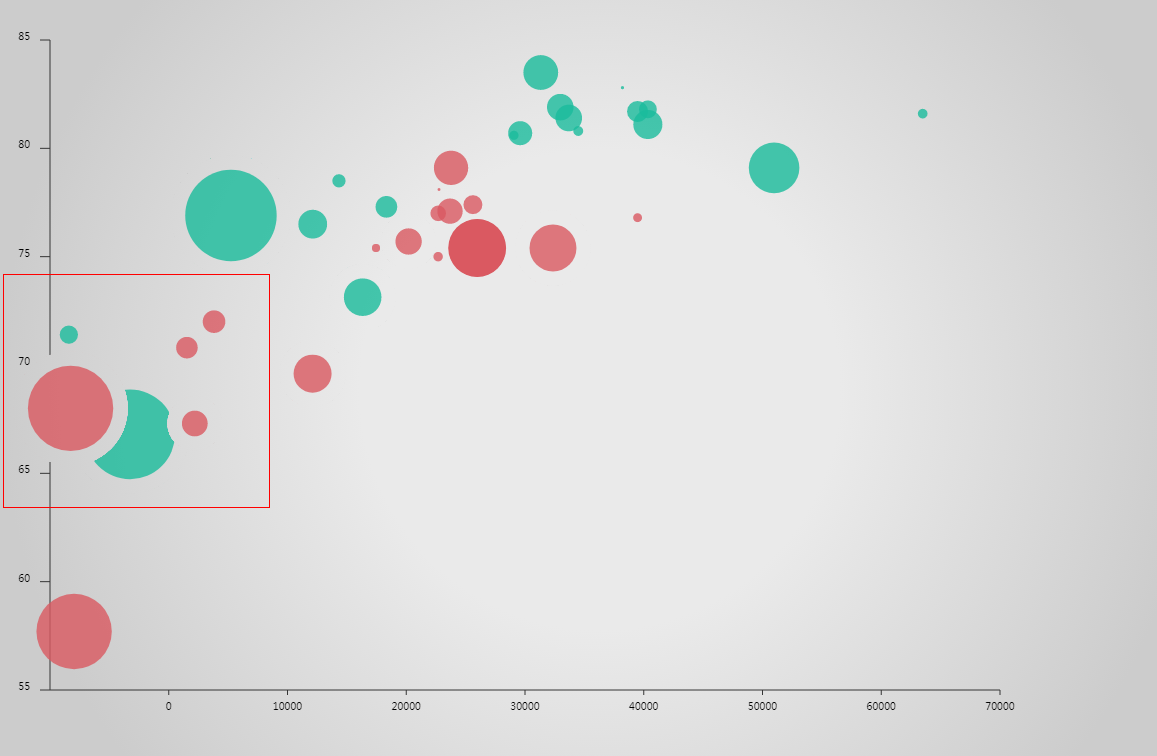posted @ 2019-03-24 20:06  大史不说话  阅读(1196)  评论(0编辑  收藏